﻿ Texture Generation

# Procedural Texture GenerationIan Parberry, "The Unexpected Beauty of Modular Bivariate Quadratic Functions", Journal of Mathematics and the Arts, Vol. 12, No. 4, pp. 197-206, 2018.

### Abstract

Modular bivariate quadratic functions are used to procedurally generate textures that resemble ornamentation, skin, scales, feathers, and textiles. They are easy to implement and particularly suited to parallel execution as a pixel shader. A prototype browser-based procedural texture generator with an interface suitable for use by non-mathematicians such as designers and artists is described.

## Biq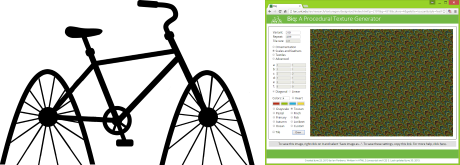Biq is a browser-enabled design tool for creating procedural textures using modular bivariate quadratic functions. It is implemented in HTML 5, CSS 3, and JavaScript.

## Images from the Manuscript

The following are larger versions of the textures from the figures in the manuscript. Click on the images to open in Biq.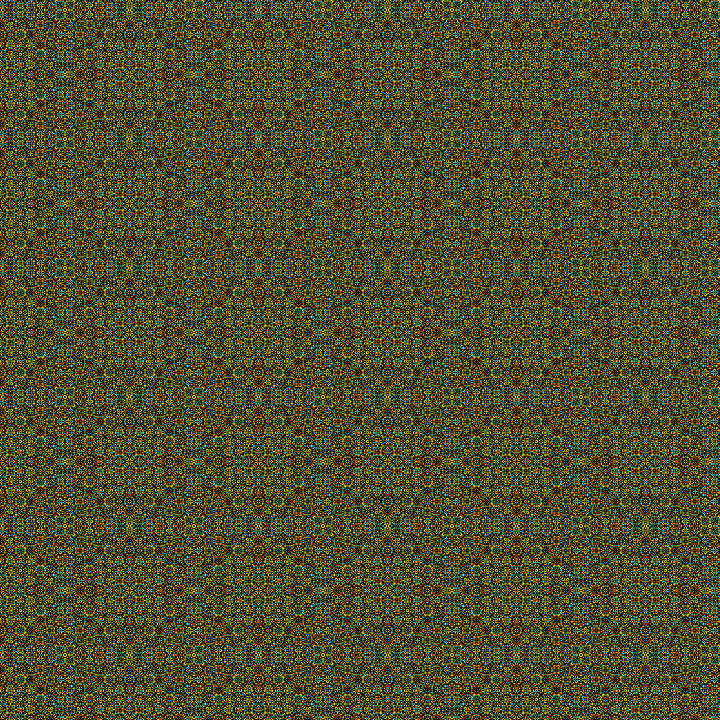Figure 1: Rectilinear ornamentation, p=38, q=129.Figure 3 (left): Rectilinear ornamentation, p=13, q=131.Figure 3 (center): Rectilinear ornamentation, p=15, q=131.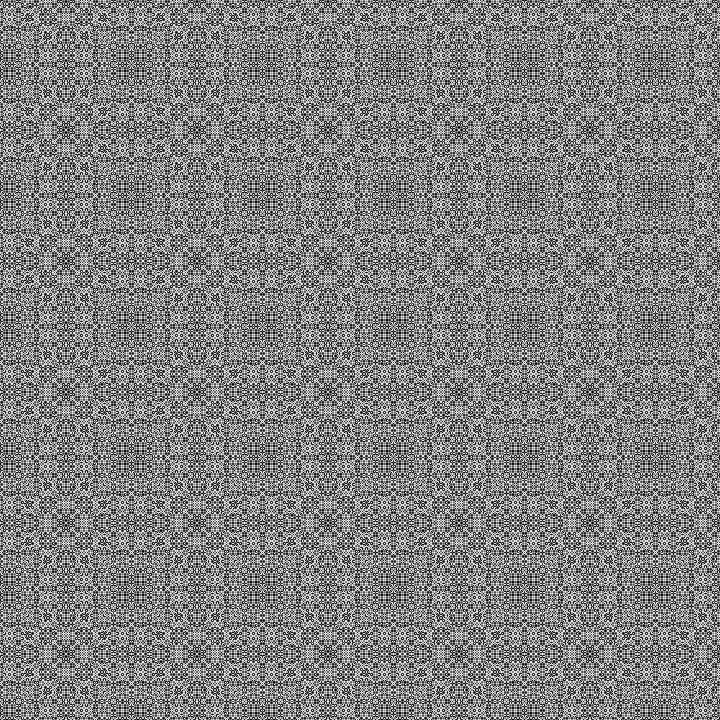Figure 3 (right): Rectilinear ornamentation, p=22, q=131.Figure 4 (left): Rectilinear ornamentation, p=22, q=131, 2 grayscale bands.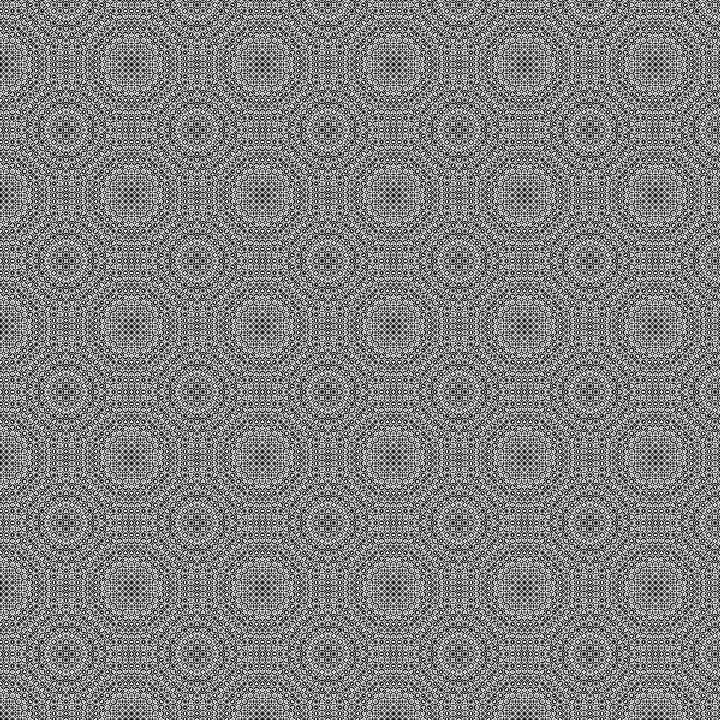Figure 4 (center): Rectilinear ornamentation, p=22, q=131, 3 grayscale bands.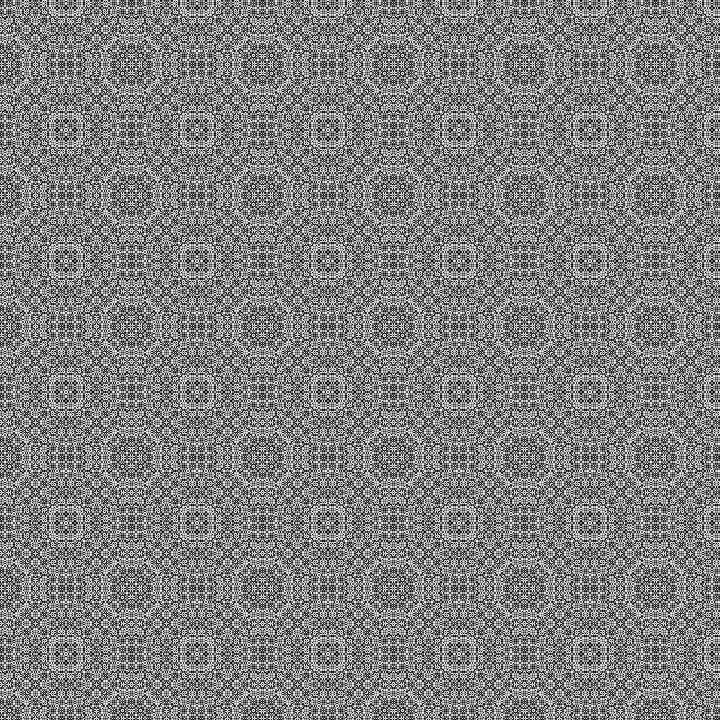Figure 4 (right): Rectilinear ornamentation, p=22, q=131, 4 grayscale bands.Figure 5 (left): Rectilinear ornamentation, p=4, q=101, 4 color bands, Primary palette.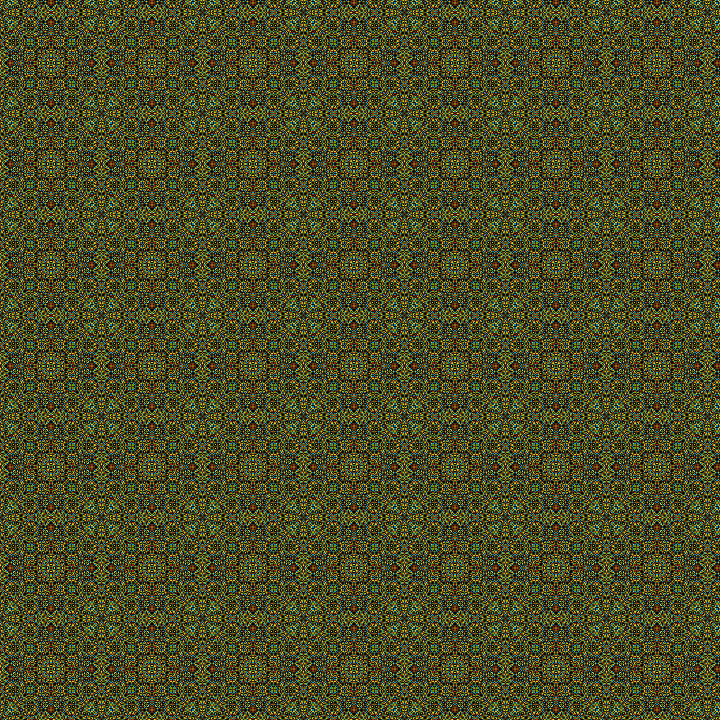Figure 5 (center): Rectilinear ornamentation, p=4, q=101, 4 color bands, Toucan palette.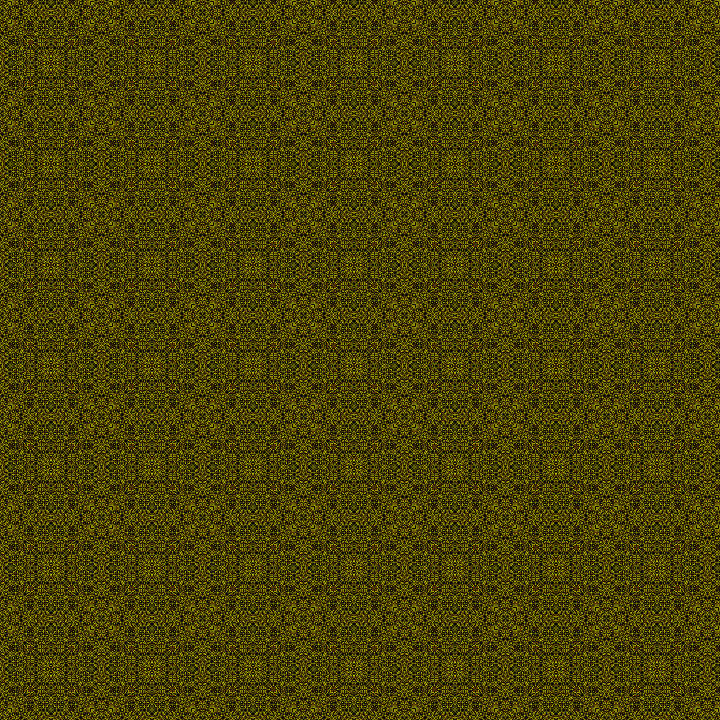Figure 5 (right): Rectilinear ornamentation, p=4, q=101, 4 color bands, Autumn palette.Figure 6 (left): Diagonal ornamentation, p=11, q=131.Figure 6 (center): Diagonal ornamentation, p=46, q=131.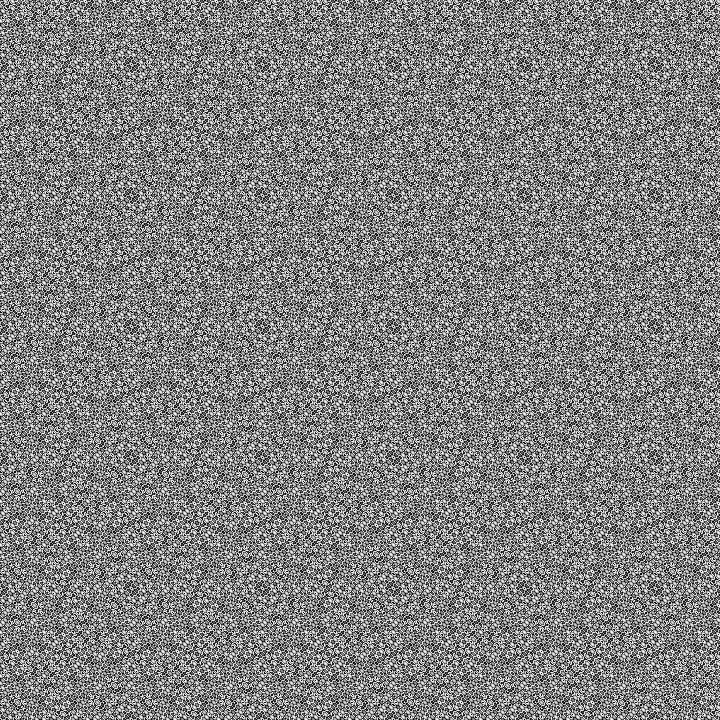Figure 6 (right): Diagonal ornamentation, p=47, q=131.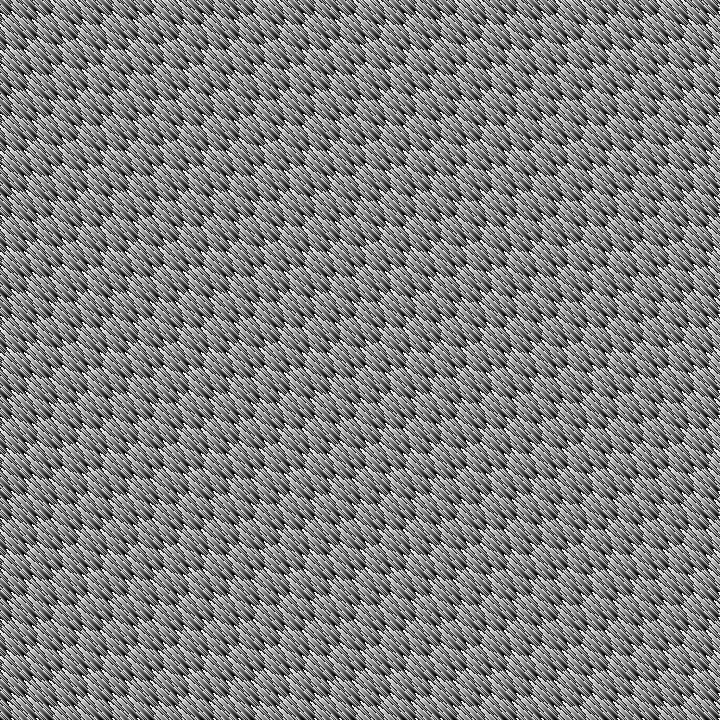Figure 7 (left): Scales and feathers, p=2, q=101.Figure 7 (center): Scales and feathers, p=3, q=101.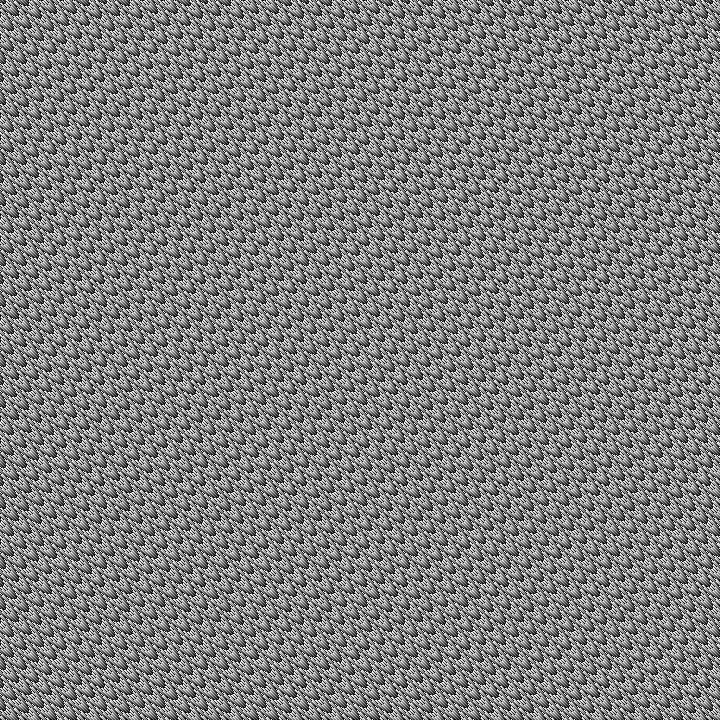Figure 7 (right): Scales and feathers, p=4, q=101.Figure 8 (left): Scales and feathers, p=10, q=101.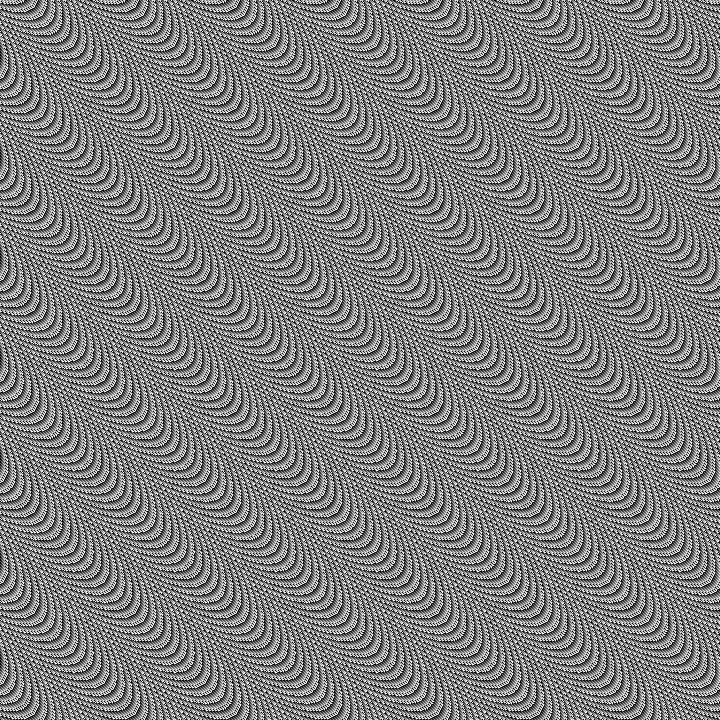Figure 8 (center): Scales and feathers, p=44, q=101.Figure 8 (right): Scales and feathers, p=45, q=101.Figure 10 (left): Textile, p=8, q=256.Figure 10 (center): Textile, p=38, q=256.Figure 10 (right): Textile, p=136, q=256.Figure 11 (left): Textile, p=5260, q=26509, 4 color bands, Ocean palette.Figure 11 (center): Scales and feathers, p=11, q=217, 4 color bands, Autumn palette.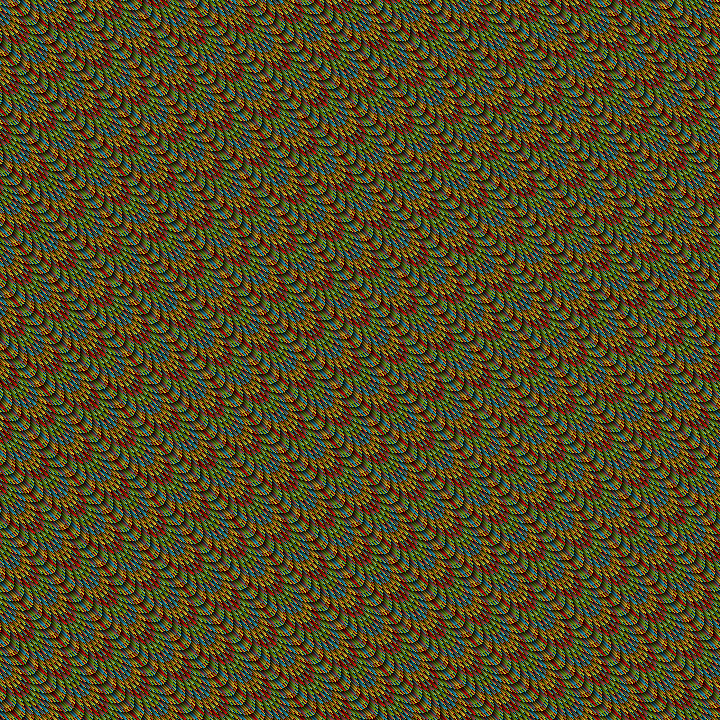Figure 11 (right): Scales and feathers, p=2101, q=4319, 4 color bands, Toucan palette.

## Supplementary ImagesCreated June 15, 2015. Last updated September 24, 2019.